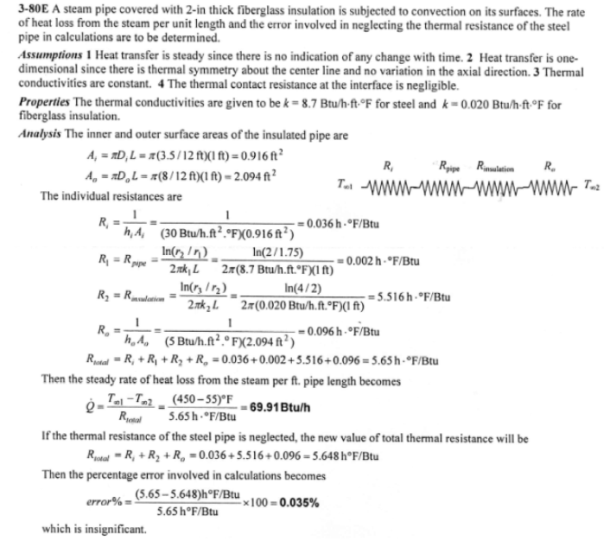heat and mass transfer problems engineering equations heat and mass transfer school homework engineering heat and mass transfer formulas heat and mass transfer solutions to heat and mass transfer problems full solution engineering problem solution heat and mass transfer math problems engineering equations heat and mass transfer school homework engineering solutions to heat and mass transfer formulas heat problems mass problem solutions to transfer problems full solution heat and mass transfer
heat and mass transfer problems engineering equations heat and mass transfer school homework engineering heat and mass transfer formulas heat and mass transfer solutions to heat and mass transfer problems full solution engineering problem solution heat and mass transfer math problems engineering equations heat and mass transfer school homework engineering solutions to heat and mass transfer formulas heat problems mass problem solutions to transfer problems full solution heat and mass transfer
Highalphabet Home Page heat and mass transfer problem solutions Heat and Mass Transfer Page
Steam at 450 F is flowing through a steel pipe (k=8.7 Btu/h ft F) whose inner and outer diameters are 3.5 in and 4.0 in, respectively, in an environment at 55 F. The pipe is insulated with 2-in-thick fiberglass insulation (k=0.020 Btu/h ft F). If the heat transfer coefficients on the inside and the outside of the pipe are 30 and 5 Btu/ h ft^2 F, respectively, determine the rate of heat loss from the steam per foot length of the pipe. What is the error involved in neglecting the thermal resistance of the steel pipe in calculations?Steam at 450 F is flowing through a steel pipe (k=8.7 Btu/h ft F) whose inner and outer diameters are 3.5 in and 4.0 in, respectively, in an environment at 55 F. The pipe is insulated with 2-in-thick fiberglass insulation (k=0.020 Btu/h ft F). If the heat transfer coefficients on the inside and the outside of the pipe are 30 and 5 Btu/ h ft^2 F, respectively, determine the rate of heat loss from the steam per foot length of the pipe. What is the error involved in neglecting the thermal resistance of the steel pipe in calculations?